Next: Problems with the interval-valuation Up: Interval Analysis Previous: Mathematical background   Contents

Implementation

All the procedures described in the following sections use the free interval analysis package BIAS/Profil in which the basic operations of interval analysis are implemented2.1.

This package uses a fixed precision arithmetics with an accuracy of roughly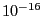. Different types of data structure are implemented in this package. For fixed value number:

BOOL, BOOL_VECTOR, BOOL_MATRIX,
INT, INTEGER_VECTOR, INTEGER_MATRIX, REAL, VECTOR, MATRIX, COMPLEX
for intervals:

INTERVAL, INTERVAL_VECTOR, INTERVAL_MATRIX
All basic arithmetic operations can be used on interval-valued data using the same notation than for fixed numbers. Not that for vector and matrices the index start at 1: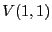for an interval matrix represents the interval at the first row and first column of the interval matrix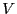. The type INTERVAL_VECTOR will be used to implement the box concept, while the type INTERVAL_MATRIX will be used to implement the concept of list of boxes.

For the evaluation of more complex interval-valued function there are also equivalent function in the BIAS/Profil, whose name is usually obtained from their equivalent in the C language by substituting their first letter by the equivalent upper-case letter: for example the evaluation of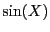where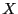is an interval will be obtained by calling the function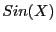. We have also introduced in ALIAS some other mathematical operators whose names are derived from their Maple implementation: ceil, floor, round.

Table 2.1 indicates the substitution for the most used functions.

Table 2.1: Equivalent interval-valued function
 C function Substitution C function Substitution sin Sin cos Cos tan Tan arcsin ArcSin arccos ArcCos arctan ArcTan sinh Sinh cosh Cosh tanh Tanh arcsinh ArcSinh arccosh ArcCosh arctanh ArcTanh exp Exp log Log log10 Log10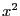Sqr sqrt Sqrt(x)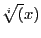Root(x,i)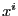Power(x,i)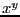Power(x,y)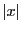IAbs(x) ceil(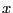) ALIAS_Ceil() floor() ALIAS_Floor() rint() ALIAS_Round()

Note also that the mathematical operators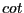,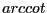,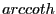exist under the name Cot, ArcCot, ArCoth.

A special operator is defined in the procedure ALIAS_Signum: formally it defines the signum operator of Maple defined as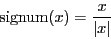which is not defined at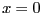. In our implementation for an interval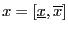ALIAS_Signum() will return:
$$1if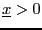$$
-1 if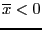$$1ifis lower than ALIAS_Value_Sign_Signum and ALIAS_Sign_Signum is positive$$
-1 ifis lower than ALIAS_Value_Sign_Signum and ALIAS_Sign_Signum is negative
-1,1
otherwise
The default value for ALIAS_Value_Sign_Signum and ALIAS_Sign_Signum are respectively 1e-6 and 0.

The derivative of ALIAS_Signum is defined in the procedure ALIAS_Diff_Signum. Formally this derivative is 0 for anynot equal to 0. In our implementation ALIAS_Diff_Signum() will return 0 except ifis lower than ALIAS_Value_Sign_Signum in which case the procedure returns [-1e11,1e11].

The derivative of the absolute value is defined in the procedure ALIAS_Diff_Abs. If the interval X includes 0 the procedure returns [-1e11,1e11] otherwise it returns ALIAS_Signum(X).

Using the above procedures when an user has to write an interval-valued function he has to convert its C source code using the defined substitution. For example if a function is written in C as: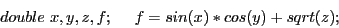then its equivalent interval valued function is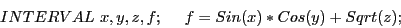Note that all the even powers of an interval are better managed with the Sqr and Power procedures. Indeed let consider the interval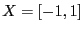, then the interval product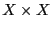leads to the interval [-1,1] while the interval Sqr() leads to [0,1]. For an interval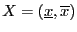the width of the interval is obtained by using the procedure Diam() while we have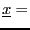Inf() and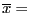Sup().

We will denote by box a set of intervals which define the possible values of the unknowns. By extension and according to the context boxes may also be used to denote a set of such set. A function intervals will denote the interval values of a set of functions for a given box, while a solution intervals will denote the box which are considered to be solution of a system of functions.Next: Problems with the interval-valuation Up: Interval Analysis Previous: Mathematical background   Contents
Jean-Pierre Merlet 2012-12-20